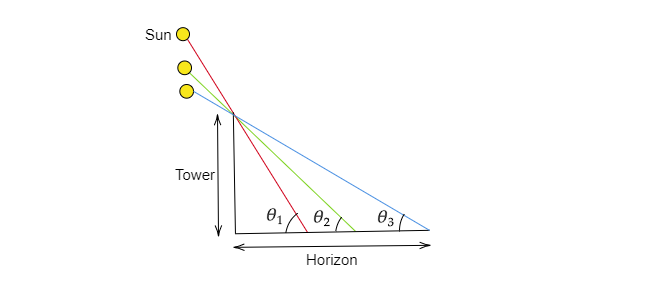# If the length of the shadow of a tower is increasing, then find the changes in the angle of elevation of the sun.

Given: The length of the shadow of a tower is increasing.

To do: To find the changes in the angle of elevation of the sun.

Solution:We can express the angle of elevation as $tan\theta=\frac{height of the tower}{length of the shadow}$

$\therefore tan\theta$ get decreased as the the length of the shadow increases i.e $\theta$ get decreased.

In figure it has been shown that how the angle of elevation is decreasing with the increment in the length of the shadow.

Updated on: 10-Oct-2022

26 Views